# Quartiles## Quartiles

Quartiles are the values that divide the data arranged in ascending or descending order into four equal parts. A distribution can be divided into four equal parts by three quartiles.

********************

10 Math Problems officially announces the release of Quick Math Solver, an Android App on the Google Play Store for students around the world.

********************

-      The first or lower quartile (Q1) is the point below which 25% of the items lie and above which 75% of the items lie.

-      The second quartile (Q2) is the point below which 50% of the items lie and above which 50% of the items lie. Of course, the second quartile is the median.

-      The third or upper quartile (Q3) is the point below which 75% of the items lie and above which, 25% of the items lie.If N is the number of items in a distribution (data) arranged in ascending or descending order, then

In the case of the individual and discrete data,In case of the grouped or continuous data,Where,

L = lower limit of the quartile class

f = frequency of the quartile class

cf = cumulative frequency of preceding class

i = height of class-interval

### Worked Out Examples

Example 1: Calculate the quartiles from the data: 4, 6, 5, 9, 8, 10, 3

Solution: Here,

Data in ascending order: 3, 4, 5, 6, 8, 9, 10

No. of data (N) = 7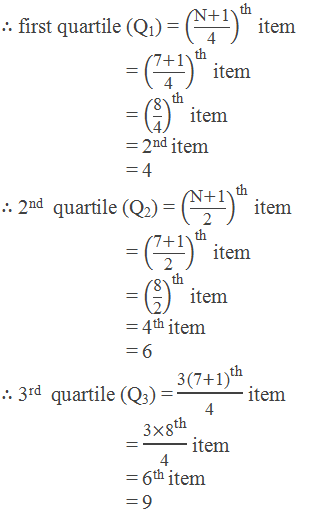Q1 = 4, Q2 = 6, and Q3 = 9 Ans.

Example 2: Calculate the quartiles from the data: 5, 8, 10, 11, 12, 14, 16, 18, 20, 4

Solution: Here,

Data in ascending order: 4, 5, 8, 10, 11, 12, 14, 16, 18, 20

No. of data (N) = 10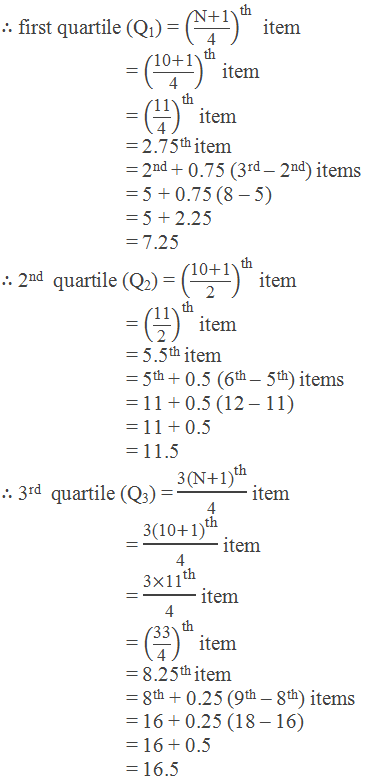Q1 = 7.25, Q2 = 11.5, and Q3 = 16.5 Ans.

Example 3: Compute Q1 and Q3 from the following data.Solution: Here,

Frequency distribution table,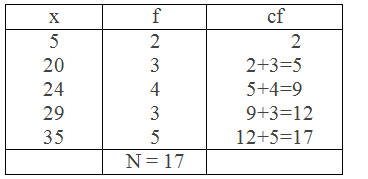Total number of data (N) = 17Q1 = 20 and Q3 = 35 Ans.

Example 4: Compute Q1 and Q3 from the following data.Solution: Here,

Frequency distribution table,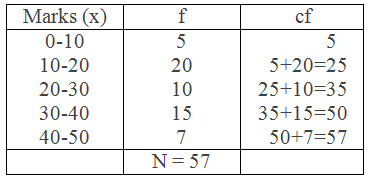Here, N = 57Q1 = 14.62 and Q3 = 35.16 Ans.

If you have any questions or problems regarding the Quartiles, you can ask here, in the comment section below.

1.Practical use of Quartile in real life?

1.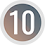Quartiles and IQR (Inter Quartile Range) are used by companies to benchmark ourselves and the other companies. For example, the pay scale for a certain position is determined on the basis of the benchmark of the company which is measured on the basis of quartiles of different data related to the company.

It is also used for the measure of sells and traffic of any product, website, web application, software, mobile app etc.

2.Hello :D! I have a question, why in the final exercise Q3 you wrote 10/15?, just that thank you.

1.Replacing the values of i and f just from above.

3.in example 4
how we know valus of cf,L and i

1.After finding the quartile class,

L = lower limit of the quartile class
f = frequency of the quartile class
cf = cumulative frequency of preceding class
i = height of class-interval

2.If i is the height of class interval then, it must be 11 instead of 10.

4.Shouldn't it be the last intervel in the last question